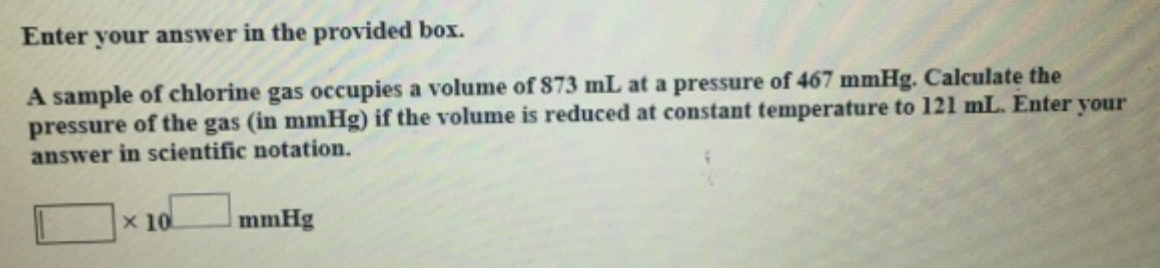# Problem: A sample of chlorine gas occupies a volume of 873 mL at a pressure of 467 mmHg. Calculate the pressure of the gas (in mmHg) if the volume is reduced at constant temperature to 121 mL. Enter your answer in scientific notation.

###### FREE Expert Solution###### Problem Details

A sample of chlorine gas occupies a volume of 873 mL at a pressure of 467 mmHg. Calculate the pressure of the gas (in mmHg) if the volume is reduced at constant temperature to 121 mL. Enter your answer in scientific notation.